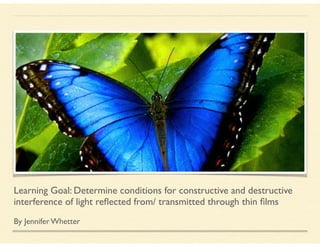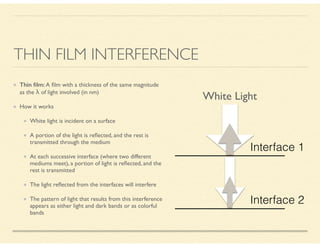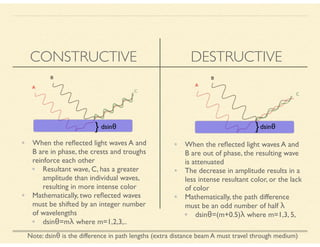Successfully reported this slideshow.×
1 of 8

Thin Film Interference Jennifer Whetter

1

Share

This LO presents conditions for constructive and destructive thin film interference. An example of thin film interference in butter fly wings with a worked solution is provided to assist in application of the concepts and demonstrate the real life applications of this topic.

See all

See all

Thin Film Interference Jennifer Whetter

1. 1. Learning Goal: Determine conditions for constructive and destructive interference of light reﬂected from/ transmitted through thin ﬁlms ! By Jennifer Whetter
2. 2. THIN FILM INTERFERENCE Thin ﬁlm: A ﬁlm with a thickness of the same magnitude as the λ of light involved (in nm) How it works White light is incident on a surface A portion of the light is reﬂected, and the rest is transmitted through the medium At each successive interface (where two different mediums meet), a portion of light is reﬂected, and the rest is transmitted The light reﬂected from the interfaces will interfere The pattern of light that results from this interference appears as either light and dark bands or as colorful bands Interface 1 Interface 2 White Light
3. 3. CONSTRUCTIVE DESTRUCTIVE • When the reﬂected light waves A and B are in phase, the crests and troughs reinforce each other • Resultant wave, C, has a greater amplitude than individual waves, resulting in more intense color • Mathematically, two reﬂected waves must be shifted by an integer number of wavelengths • dsinθ=mλ where m=1,2,3,.. • When the reﬂected light waves A and B are out of phase, the resulting wave is attenuated • The decrease in amplitude results in a less intense resultant color, or the lack of color • Mathematically, the path difference must be an odd number of half λ • dsinθ=(m+0.5)λ where m=1,3, 5, } dsinθ }dsinθ Note: dsinθ is the difference in path lengths (extra distance beam A must travel through medium)
4. 4. HOWTO APPLYTHE CONCEPTS The type of interference that occurs depends on: The path length difference Whether the reﬂected waves are out of phase depends on the extra distance (through the ﬁlm and back) that the second ray must travel before rejoining the ﬁrst ray A path length difference equal to an odd number of half wavelengths results in a phase shift of π radians Reﬂection A wave is reﬂected π radians out of phase when it tries to enter a medium with a lower speed of light (the refractive index of current medium is less than the refractive index of medium it is trying to enter) Tips: You have to be very careful to account for whether a phase shift occurs at an interface where reﬂection is taking place Wavelengths (and hence interference patterns) often depend on whether or not a phase shift occurs at both, one, or none of the interfaces
5. 5. PRACTICE PROBLEM A butterﬂy’s wings are made up of two thin layers of a transparent substance with a refraction index of 1.56 called keratin.When viewed in daylight, a portion of the wings reﬂects blue light of wavelength 475nm. Estimate the minimum thickness of this section of the ﬁlm assuming m=1. Morpho menelaus
6. 6. SOLUTION Understanding the problem As the refractive index of keratin is greater than the refractive index (n) of air, ray 2 will be reﬂected out of phase with ray 1 (with a phase inversion of 1/2 a λ) At the second interface, the ray is traveling from keratin to air (higher to lower refractive index), and ray 5 will be in phase with ray 1 Since one reﬂection is 1/2 λ and the other is 0 λ, the net phase inversion, Φ is 1/2 λ Since bright blue is observed, you are dealing with constructive interference therefore you use the formula: path difference=mλ where m=1,2,3
7. 7. Solving the problem Find the wavelength of blue light in keratin The λ of blue light is 475nm in air Therefore, λkeratin=λair/nkeratin=475/1.56=304nm Equation for thin ﬁlm interference path difference=2t+Φ where m=1,2,3,… mλ =2t+1/2λ where t is thickness 2t=mλkeratin-1/2λkeratin t=1/2(m-1/2)(304) t=1/2(1-1/2)(304) t=1/4(304) t=76nm SOLUTION CONTINUED minimum non-zero thickness of keratin ﬁlm
8. 8. REFERENCES http://www.webexhibits.org/causesofcolor/15E.html http://physics.bu.edu/py106/notes/Thinﬁlm.html http://www.tufts.edu/as/tampl/projects/micro_rs/theory.html http://dev.physicslab.org/Document.aspx? doctype=3&ﬁlename=PhysicalOptics_ThinFilmInterference.xml